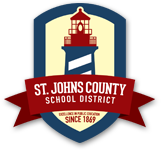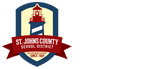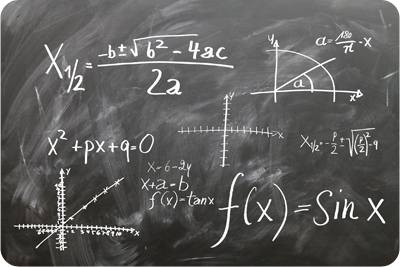• News
• Careers
• Calendar
• Home Access Center (HAC)
• Contact

# + Positive + Start Program

Math is fun, but it can also be challenging.

The + Positive + Start Program enables students to practice the prerequisite math skills needed to be successful in the mathematics course in which they are enrolled in the next school year.

Completion of the course will likely give students a positive start in math.

It’s never too late for a positive start. This program will be available to students through the fall and will end December 31, 2021.

The +Positive + Start Program supports the prerequisite skills needed for the mathematics courses listed below:Mathematics Course

Prerequisite Mathematics Skills Review

• Get ready for ratios, rates, and percentages
• Get ready for arithmetic operations
• Get ready for negative numbers
• Get ready for equations, expressions, and inequalities

Code: ANDRKGEH

• Get ready for negative number operations
• Get ready for fractions, decimals, & percentages
• Get ready for rates & proportional relationships
• Get ready for expressions, equations, & inequalities
• Get ready for statistics & probability

Code: 5S2J63BQ

• Get ready for numbers and operations
• Get ready for solving equations and systems of equations
• Get ready for linear equations and functions

Code: MWWFFWAU

Algebra 1

• Get ready for equations & inequalities
• Get ready for working with units
• Get ready for linear relationships
• Get ready for functions & sequences

Code: A7ZENQUK

Geometry

• Get ready for congruence, similarity, and triangle trigonometry
• Get ready for analytic geometry
• Get ready for conic sections
• Get ready for circles and solid geometry

Code: 7YUVHHDG

Algebra 2

• Get ready for polynomial operations and complex numbers
• Get ready for transformations of functions and modeling with functions
• Get ready for exponential and logarithmic relationships
• Get ready for rational functions

Code: VFXCBC38

Pre-Calculus Honors

• Modeling (Algebra 2)
• The unit circle definition of sine, cosine, and tangent (Trigonometry)
• Graphs of trigonometric functions (Trigonometry)
• Trigonometric equations and identities (Trigonometry)

Code: 48BQSZQN

AP Calculus

• Modeling (Algebra 2)
• The unit circle definition of sine, cosine, and tangent (Trigonometry)
• Graphs of trigonometric functions (Trigonometry)
• Trigonometric equations and identities (Trigonometry)

Code: TSUHZHF5